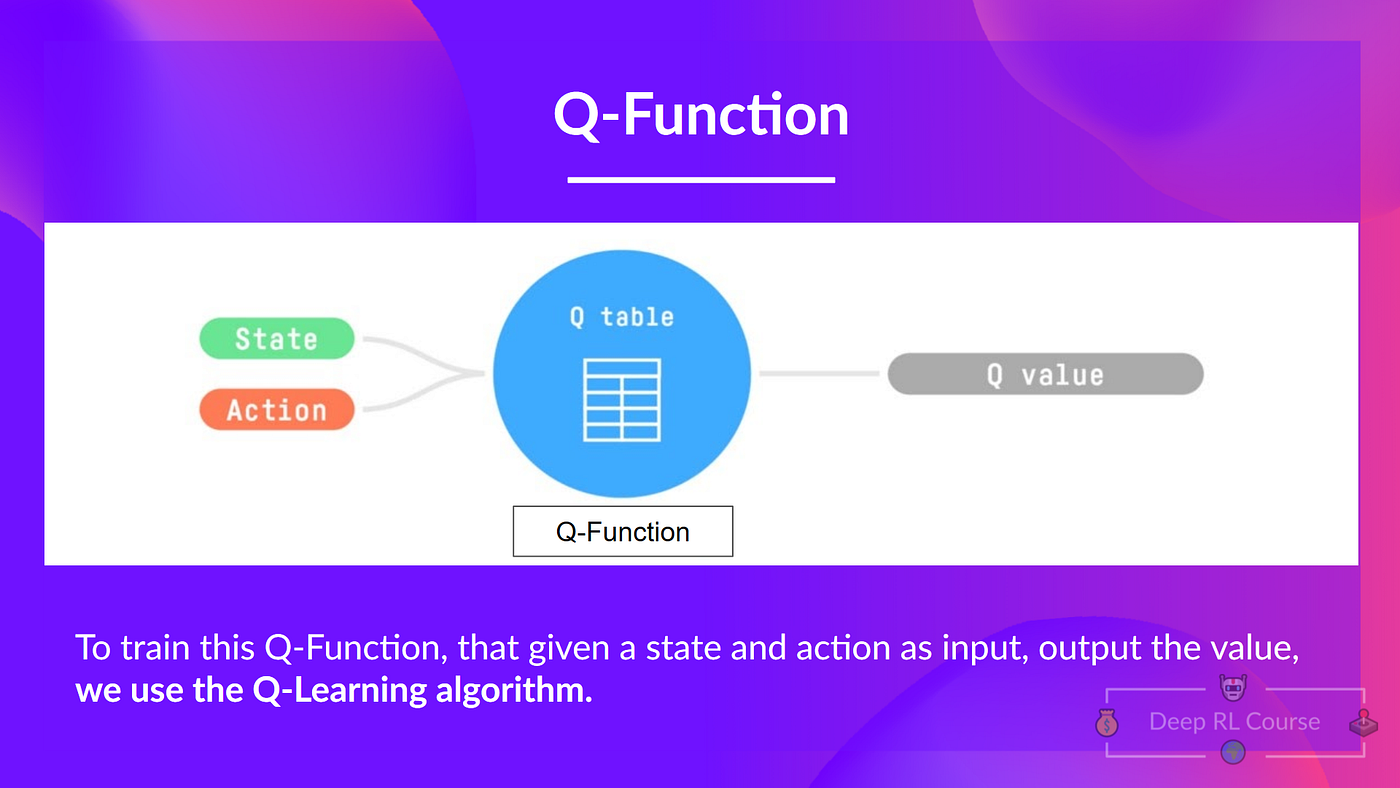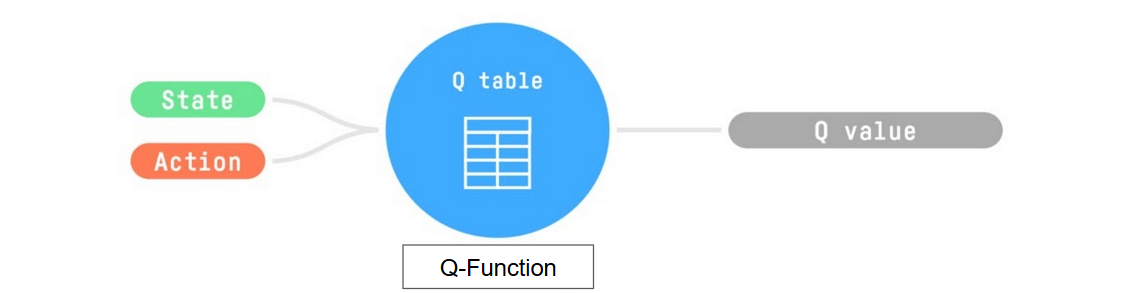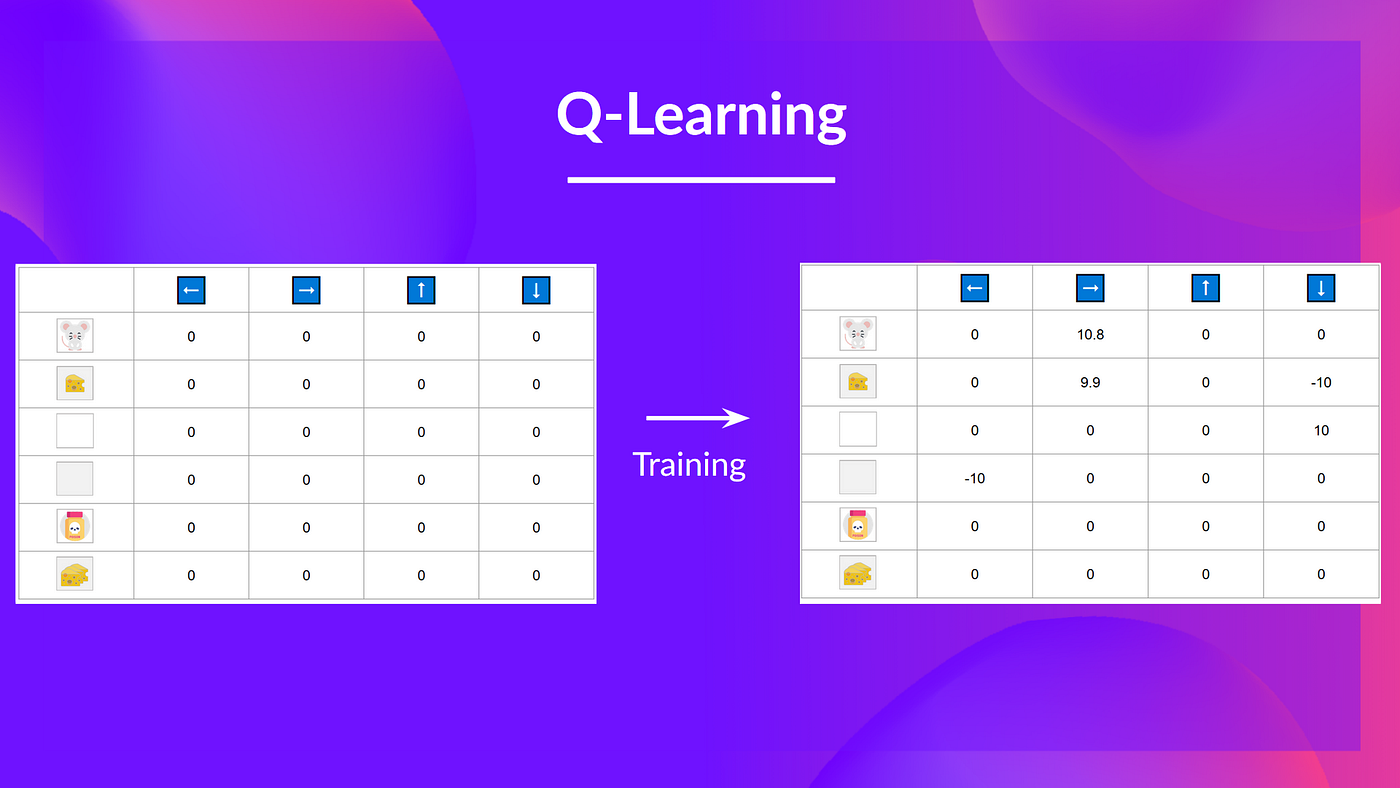# Q-Learning, let’s create an autonomous Taxi 🚖 (Part 2/2)

We launched a new free, updated, Deep Reinforcement Learning Course from beginner to expert, with Hugging Face 🤗

👉 Register here http://eepurl.com/h1pElX

The chapter below is the former version, the new version is here 👉 https://huggingface.co/blog/deep-rl-q-part2

We launched a new free, updated, Deep Reinforcement Learning Course from beginner to expert, with Hugging Face 🤗

👉 Register here http://eepurl.com/h1pElX

The chapter below is the former version, the new version is here 👉 https://huggingface.co/blog/deep-rl-q-part2

In the first part of this second chapter of this course, we learned about the value-based methods and the difference between Monte Carlo and Temporal Difference Learning.

So, in the second part, we’ll study Q-Learning, and implement our first RL Agent: a Q-Learning autonomous taxi that will need to learn to navigate in a city to transport its passengers from point A to point B.

This chapter is fundamental if you want to be able to work on Deep Q-Learning (chapter 3): the first Deep RL algorithm that was able to play Atari games and beat the human level on some of them (breakout, space invaders…).

So let’s get started!

# Introducing Q-Learning

## What is Q-Learning?

Q-Learning is an off-policy value-based method that uses a TD approach to train its action-value function:

• “Off-policy”: we’ll talk about that at the end of this chapter.
• “Value-based method”: it means that it finds its optimal policy indirectly by training a value-function or action-value function that will tell us what’s the value of each state or each state-action pair.
• “Uses a TD approach”: updates its action-value function at each step.

In fact, Q-Learning is the algorithm we use to train our Q-Function, an action-value function that determines the value of being at a certain state, and taking a certain action at that state.Given a state and action, our Q Function outputs a state-action value (also called Q-value)

The Q comes from “the Quality” of that action at that state.

Internally, our Q-function has a Q-table, which is a table where each cell corresponds to a state-action value pair value. Think of this Q-table as the memory or cheat sheet of our Q-function.

If we take this maze example:

The Q-Table (just initialized that’s why all values are = 0), contains for each state, the 4 state-action values.

Here we see that the state-action value of the initial state and going up is 0:

Therefore, Q-Function contains a Q-table that contains the value of each-state action pair. And given a state and action, our Q-Function will search inside its Q-table to output the value.Given a state and action pair, our Q-function will search inside its Q-table to output the state-action pair value (the Q value).

So, if we recap:

• The Q-Learning is the RL algorithm that
• Trains Q-Function, an action-value function that contains, as internal memory, a Q-table that contains all the state-action pair values.
• Given a state and action, our Q-Function will search into its Q-table the corresponding value.
• When the training is done, we have an optimal Q-Function, so an optimal Q-Table.
• And if we have an optimal Q-function, we have an optimal policy, since we know for each state, what is the best action to take.

But, in the beginning, our Q-Table is useless since it gives arbitrary value for each state-action pair (most of the time we initialize the Q-Table to 0 values). But, as we’ll explore the environment and update our Q-Table it will give us better and better approximations.We see here that with the training, our Q-Table is better since thanks to it we can know the value of each state-action pair.

So now that we understood what are Q-Learning, Q-Function, and Q-Table, let’s dive deeper into the Q-Learning algorithm

## The Q-Learning algorithm

This is the Q-Learning pseudocode, let’s study each part, then we’ll see how it works with a simple example before implementing it.

Step 1: We initialize the Q-Table

We need to initialize the Q-Table for each state-action pair. Most of the time we initialize with values of 0.

Step 2: Choose action using Epsilon Greedy Strategy

Epsilon Greedy Strategy is a policy that handles the exploration/exploitation trade-off.

The idea is that we define epsilon ɛ = 1.0:

• With probability 1 — ɛ : we do exploitation (aka our agent selects the action with the highest state-action pair value).
• With probability ɛ: we do exploration (trying random action).

At the beginning of the training, the probability of doing exploration will be very big since ɛ is very high, so most of the time we’ll explore. But as the training goes, and consequently our Q-Table gets better and better in its estimations, we progressively reduce the epsilon value since we will need less and less exploration and more exploitation.

Step 3: Perform action At, gets Rt+1 and St+1

Step 4: Update Q(St, At)

Remember that in TD Learning, we update our policy or value function (depending on the RL method we choose) after one step of interaction.

To produce our TD target, we used the immediate reward Rt+1 plus the discounted value of the next state best state-action pair (we call that bootstrap).

Therefore, our Q(St, At) update formula goes like this:

It means that to update our Q(St,At):

• We need St, At, Rt+1, St+1.
• To update our Q-value at this state-action pair, we form our TD target:

We use Rt+1 and to get the best next-state-action pair value, we select with a greedy-policy (so not our epsilon greedy policy) the next best action (so the action that have the highest state-action value).

Then when the update of this Q-value is done. We start in a new_state and select our action using our epsilon-greedy policy again.

It’s why we say that this is an off-policy algorithm.

## Off-policy vs On-policy

The difference is subtle:

• Off-policy: using a different policy for acting and updating.

For instance, with Q-Learning, the Epsilon greedy policy (acting policy), is different from the greedy policy that is used to select the best next-state action value to update our Q-value (updating policy).

Is different from the policy we use during the training part:

• On-policy: using the same policy for acting and updating.

For instance, with Sarsa, another value-based algorithm, it’s the Epsilon-Greedy Policy that selects the next_state-action pair, not a greedy-policy.

## An example

To better understand this algorithm, let’s take a simple example:

• You’re a mouse in this very small maze. You always start at the same starting point.
• The goal is to eat the big pile of cheese at the bottom right-hand corner, and avoid the poison.
• The episode ends if we eat the poison, eat the big pile of cheese or if we spent more than 5 steps.
• The learning rate is 0.1
• The gamma (discount rate) is 0.99

The reward function goes like this:

• +0: Going to a state with no cheese in it.
• +1: Going to a state with a small cheese in it.
• +10: Going to the state with the big pile of cheese.
• -10: Going to the state with the poison and thus die.

To train our agent to have an optimal policy (so a policy that goes left, left, down). We will use the Q-Learning algorithm.

Step 1: We initialize the Q-Table

So, for now, our Q-Table is useless, we need to train our Q-Function using Q-Learning algorithm.

Let’s do it for 2 steps:

Step 2: Choose action using Epsilon Greedy Strategy

Because epsilon is big = 1.0, I take a random action, in this case I go right.

Step 3: Perform action At, gets Rt+1 and St+1

By going right, I’ve got a small cheese so Rt+1 = 1 and I’m in a new state.

Step 4: Update Q(St, At)

We can now update Q(St, At) using our formula.

STEP 2:

Step 2: Choose action using Epsilon Greedy Strategy

I take again a random action, since epsilon is really big 0.99 (since we decay it a little bit because as the training progress we want less and less exploration).

I took action down. Not a good action since it leads me to the poison.

Step 3: Perform action At, gets Rt+1 and St+1

Because I go to the poison state, I get Rt+1 = -10 and I die.

Step 4: Update Q(St, At)

Because we’re dead, we start a new episode. But what we see here, is that with two explorations steps, my agent became smarter.

As we continue to explore and exploit the environment and update Q-values using TD target, Q-Table will give us better and better approximations. And thus, at that end of the training, we’ll get an optimal Q-Function.

We’re now ready to implement our first RL agent,

# Let’s train our Q-Learning Taxi agent 🚕

Now that we understood the theory behind Q-Learning, let’s implement our first agent.

The goal here is to train a taxi agent to navigate in this city to transport its passengers from point A to point B.

Our environment looks like this, it’s a 5x5 grid world, our taxi is spawned randomly in a square. The passenger is spawned randomly in one of the 4 possible locations (R, B, G, Y) and wishes to go in one of the 4 possibles locations too.

Your task is to pick up the passenger at one location and drop him off in its desired location (selected randomly).

There are 6 possible actions, the actions are deterministic (it means the one you choose to take is the one you take):

The reward system:

Why we set a -1 for each action?

Remember that the goal of our agent is to maximize its expected cumulative reward, if the reward is -1, its goal is to have the minimum amount possible of negative reward (since he wants to maximize the sum), so it will push him to go the faster possible. So to take the passenger from his location to its destination as fast as possible.

For this part, you can follow the notebook every is explained step by step, or watch the video version of the course where we’ll implement it step by step.

So let’s start,

So that’s all for today. Congrats on finishing this chapter!

You’ve just implemented your first RL agent, an autonomous taxi that is able to navigate in this city to transport its passengers from a random point A to a random point B. That’s amazing!

Take time to grasp the material before continuing. In the third chapter, we’ll study Deep Q-Learning, and implement our first Deep RL Agent that will learn to play Space Invaders.

If you liked my article, please click the 👏 below as many times as you liked the article so other people will see this here on Medium. And don’t forget to follow me on Medium, on Twitter, and on Youtube.

See you next time,

Keep learning, stay awesome,

--

--